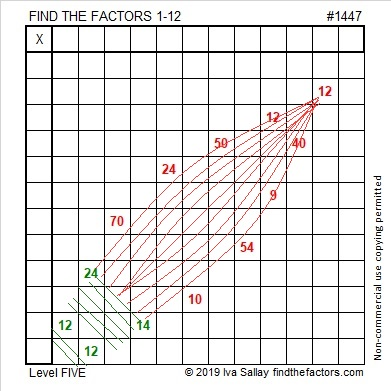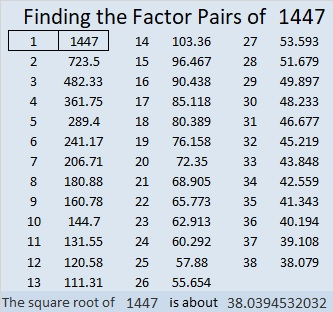# 1447 Christmas Light Puzzle

If you’ve ever had a string of lights go out because ONE bulb went bad, it can be a very frustrating puzzle to figure out which light is causing the problem.

This is not that kind of puzzle. For this one, you just need to figure out where to put the numbers from 1 to 12 in both the first column and the top row so that the given clues are the products of those numbers. There is only one solution, and if you always use logic, it will not be a frustrating puzzle to solve.I gave that puzzle the puzzle number 1447. That number won’t help you solve the puzzle, but here are some facts about it anyway:

• 1447 is a prime number.
• Prime factorization: 1447 is prime.
• 1447 has no exponents greater than 1 in its prime factorization, so √1447 cannot be simplified.
• The exponent in the prime factorization is 1. Adding one to that exponent we get (1 + 1) = 2. Therefore 1447 has exactly 2 factors.
• The factors of 1447 are outlined with their factor pair partners in the graphic below.How do we know that 1447 is a prime number? If 1447 were not a prime number, then it would be divisible by at least one prime number less than or equal to √1447. Since 1447 cannot be divided evenly by 2, 3, 5, 7, 11, 13, 17, 19, 23, 29, 31, or 37, we know that 1447 is a prime number.

1447 is also the difference of two consecutive squares:
724² – 723² = 1447